Pure and Applied Mathematics Journal
Volume 4, Issue 6, December 2015, Pages: 264-268

Homotopy Perturbation Transform Method for Solving Korteweg-DeVries (KDV) Equation

Mohannad H. Eljaily1, Tarig M. Elzaki2

1Department of Mathematic, Faculty of Sciences, Sudan University of Sciences and Technology, Khartoum, Sudan

2Mathematics Department, Faculty of Sciences and Arts-Alkamil, University of Jeddah, Jeddah, Saudi Arabia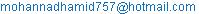(M. H. Eljaily)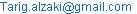(T. M. Elzaki)

Mohannad H. Eljaily, Tarig M. Elzaki. Homotopy Perturbation Transform Method for Solving Korteweg-DeVries (KDV) Equation.Pure and Applied Mathematics Journal.Vol.4, No. 6, 2015, pp. 264-268. doi: 10.11648/j.pamj.20150406.17

Abstract: In this paper, a combined form of the Laplace transforms method with the homotopy perturbation method is proposed to solve Korteweg-DeVries (KDV) Equation. This method is called the homotopy perturbation transform method (HPTM). The (HPTM) finds the solution without any discretization or restrictive assumptions and avoids the round-off errors. The results reveal that the proposed method is very efficient, simple and can be applied to other nonlinear problems.

Keywords: Laplace Transform, Homotopy Perturbation Method, Korteweg-DeVries (KDV) Equation

Contents

1. Introduction

In the recent years, the idea of homotopy was coupled with perturbation. The fundamental work was done by He. In 1992. He  developed the homotopy perturbation method (HPM) by merging the standard homotopy and perturbation for solving various physical problems. The authors have applied this method successfully to problems arising in mathematics engineering. The KDV equation plays an important role in diverse areas of engineering and scientific applications, and therefore, the enormous amount of research work has been invested in the study of KDV equations . The Laplace transform is totally incapable of handling nonlinear equations because of the difficulties that are caused by the nonlinear terms. Various ways have been proposed recently to deal with these nonlinearities such as the Adomian decomposition method  and the Laplace decomposition algorithm . Furthermore, the homotopy perturbation method is also combined with the well-known Laplace transform method  and the variational iteration method  to produce a highly effective technique for handling many nonlinear problems. In this paper, we shall deal with the KDV equation in different forms by the homotopy perturbation transform method (HPTM). The KDV equation can be presented in the following form,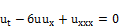(1)

where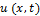is the displacement.

The purpose of this paper is to extend the (HPTM) for the solution of Korteweg-DeVries (KDV) Equation. The method has been successfully applied for obtaining exact solutions for nonlinear equations.. In this paper considers the effectiveness of the homotopy perturbation transform method in solving Korteweg-DeVries (KDV) Equation.

2. Basic Idea

To illustrate the basic idea of this method, we consider a general nonlinear non homogeneous partial differential equation with initial conditions of the form: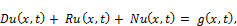(2)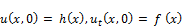Where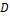is the second order linear differential operator, is the linear differential operator of less order than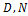represents the general non-linear differential operator and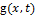is the source term.

Taking Laplace transform (denoted throughout this paper by L) on both sides of Eq. (2), to get: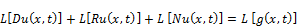(3)

Using the differentiation property of the Laplace transform, we have: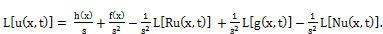(4)

Operating with the Laplace inverse on both sides of Eq. (4) gives: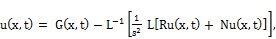(5)

whererepresents the term arising from the source term and the prescribed initial conditions. Now, we apply the homotopy perturbation method,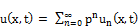(6)

and the nonlinear term can be decomposed as: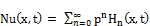(7)

for some He’s polynomials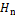(see [19,20] that are given by: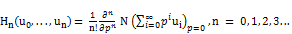(8)

Substituting Eqs. (7) and (6) in Eq. (5) we get:(9)

Which is the coupling of the Laplace transform and the homotopy perturbation method using He’s polynomials. Comparing the coefficient of like powers of p, the following approximations are obtained: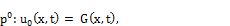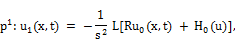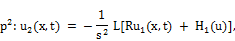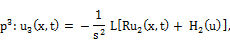Then the solution is: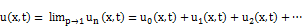(10)

3. Applications

In this section, the effectiveness and the usefulness ofhomotopyperturbationtransform method (HPTM) are demonstrated by finding exact solutions of Korteweg-DeVries (KDV) Equation.

Example 3.1. Consider the following homogeneous KDV equation;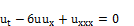(11)

With the initial condition;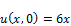(12)

Taking the Laplace transform on both sides of Eq. (11) subject to the initial

condition Eq.(12), we get;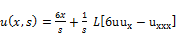(13)

The inverse of Laplace transformimplies that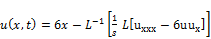(14)

Now, we apply the homotopy perturbation method, we get: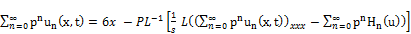(15)

Where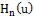are He’s polynomials that represents the nonlinear terms.

The first few components of He’s polynomials, are given by;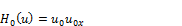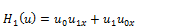(16)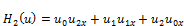Comparing the coefficient of like powers of p, the following approximations are obtained;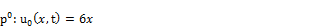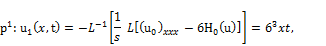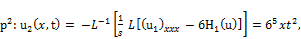(17)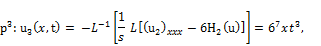Therefore the solution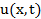is given by: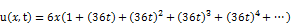(18)

In series form, and,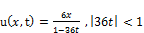(19)

In closed form.

Example 3.2. Consider the following homogeneous KDV equation;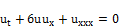(20)

With the initial condition;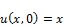(21)

Taking the Laplace transform on both sides of Eq. (20) subject to the initial

Condition Eq.(21), we get;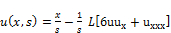(22)

The inverse of Laplace transformimplies that: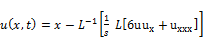(23)

Now, we apply the homotopy perturbation method, we get: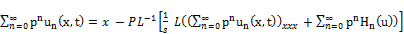(24)

Comparing the coefficient of like powers of p, the following approximations are obtained;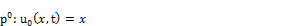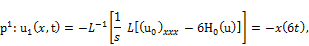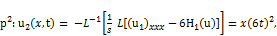(25)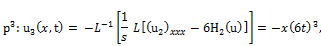Therefore the solutionis given by: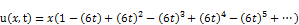(26)

In series form, and,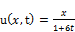(27)

In closed form.

Example 3.3. Consider the following homogeneous KDV equation;(28)

With the initial condition;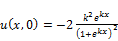(29)

Taking the Laplace transform of both sides of Eq. (28) subject to the initial

Condition Eq. (29), we get;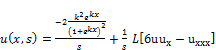(30)

The inverse of Laplace transform implies that: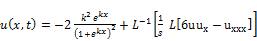(31)

Now, we apply the homotopy perturbation method, we get: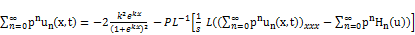(32)

Comparing the coefficient of like powers of p, the following approximations are obtained;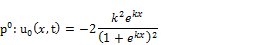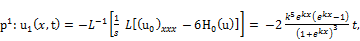(33)Therefore, the solution of Eq. (8), when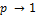will be as: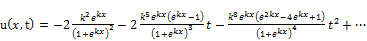(34)

Using Taylor series, the closed form solution will be as follows: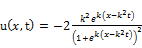(35)

4. Conclusions

In this paper, we have applied thehomotopy perturbation transform method toKorteweg-DeVries (KDV) Equation. It can be concluded that the HPTM is a very powerful and efficient technique in findingexact and approximatesolutions for nonlinear problems.Byusing this method we obtain a new efficient recurrent relation to solve (KDV) Equation. In conclusion, HPTM provide highly accurate numerical solutions for nonlinear problems in comparison with other method.The results show that the HPTM is a powerful mathematical tool for solving the KDV having wide applications in engineering and applied mathematics.

References

1. J.H. He, Homotopy perturbation technique, Computer Methods in Applied Mechanics and Engineering, 178(1999):257-262.
2. N.H. Sweilam and M.M. Khader, Exact solutions of some coupled nonlinear partial differential equations using the homotopy perturbation method, Computers & Mathematics with Applications, 58(2009):2134 2141.
3. A.M. Wazwas, A study on linear and non-linear Schrodinger equations by the variational iteration method, Chaos,Solitions and Fractals, 37(4)(2008):1136 1142.
4. B. Jazbi and M. Moini, Application of He’s homotopy perturbation method for Schrodinger equation, Iranian Journalof Mathematical Sciences and Informatics, 3(2)(2008):13-19.
5. J.H. He, Homotopy perturbation method: a new nonlinear analytical technique, Applied Mathematics and Computa- tion, 135(2003):73-79.
6. J.H. He, Comparison of homotopy perturbation method and homotopy analysis method, Applied Mathematics and Computation, 156(2004):527539.
7. J.H. He, The homotopy perturbation method for nonlinear oscillators with discontinuities, Applied Mathematics and Computation, 151(2004):287292.
8. J.H. He, Homotopy perturbation method for bifurcation of nonlinear problems, International Journal of Nonlinear Sciences and Numerical Simulation, 6(2005):207-208.
9. J.H. He, Some asymptotic methods for strongly nonlinear equation, International Journal of Modern Physics, 20(2006):1144-1199.
10. J.H. He, Homotopy perturbation method for solving boundary value problems, Physics Letters A, 350(2006):87-88.
11. Rafei and D.D. Ganji, Explicit solutions of helmhotz equation and fifth order KdV equation using homotopy perturbation method, International Journal of Nonlinear Sciences and Numerical Simulation, 7(2006):321-328.
12. A.M. Siddiqui, R. Mahmood and Q.K. Ghori, Thin film flow of a third grade fluid on a moving belt by He’s homotopy perturbation method, International Journal of Nonlinear Sciences and Numerical Simulation, 7(2006):7-14.
13. D.D. Ganji, The applications of He’s homotopy perturbation method to nonlinear equation arising in heat transfer, Physics Letters A, 335(2006):337 341.
14. L. Xu, He’s homotopy perturbation method for a boundary layer equation in unbounded domain, Computers &Math- ematics with Applications, 54(2007):1067-1070.
15. J.H. He, An elementary introduction of recently developed asymptotic methods and nanomechanics in textile engi- neering, International Journal of Modern Physics, 22(2008):3487-3578.
16. J.H. He, Recent developments of the homotopy perturbation method, Topological Methods in Nonlinear Analysis, 31(2008):205-209.
17. E. Hesameddini and H. Latifizadeh, An optimal choice of initial solutions in the homotopy perturbation method, International Journal of Nonlinear Sciences and Numerical Simulation, 10(2009):1389-1398.
18. E. Hesameddini and H. Latifizadeh, A new vision of the He’s homotopy perturbation method, International Journal of Nonlinear Sciences and Numerical Simulation, 10(2009):1415-1424.
19. A. Ghorbani and J. Saberi-Nadjafi, He’s homotopy perturbation method for calculating adomian polynomials, Inter-national Journal of Nonlinear Sciences and Numerical Simulation, 8(2007):229-232.
20. A. Ghorbani, Beyondadomian’s polynomials: He polynomials, Chaos Solitons Fractals, 39(2009):1486-1492.
21. J. Biazar, M. GholamiPorshokuhi and B. Ghanbari, Extracting a general iterative method from an adomiandecom- position method and comparing it to the variational iteration method, Computers & Mathematics with Applications, 59(2010):622-628.
22. M. Madani, M. Fathizadeh, Homotopy perturbation algorithm using Laplace transformation, Nonlinear Science Letters A 1 (2010) 263–267.
23. S.T. Mohyud-Din, M.A. Noor and K.I. Noor, Traveling wave solutions of seventh-order generalized KdV equation using He’s polynomials, International Journal of Nonlinear Sciences and Numerical Simulation, 10(2009):227-233.
24. S.A. Khuri, A Laplace decomposition algorithm applied to a class of nonlinear differential equations, Journal of Applied Mathematics 1 (2001) 141–155.
25. E. Yusufoglu, Numerical solution of Duffing equation by the Laplace decomposition algorithm, Applied Mathematics and Computation 177 (2006) 572–580.
26. Yasir Khan, An effective modification of the Laplace decomposition method for nonlinear equations, International Journal of Nonlinear Sciences and Numerical Simulation 10 (2009) 1373–1376.
27. YasirKhan,NaeemFaraz, A new approach to differential difference equations, Journal of Advanced Research in Differential Equations 2 (2010) 1–12.
28. S. Islam,Y. Khan, N. Faraz, F. Austin, Numerical solution of logistic differential equations by using the Laplace decomposition method, World AppliedSciences Journal 8 (2010) 1100–1105.
29. Dogan Kaya, Mohammed Aassila, Application for a generalized KdV equation by the decomposition method, Physics Letters A 299 (2002) 201–206.
30. P.G. Drazin, R.S. Johnson, Solutions: An Introduction, Cambridge University Press, Cambridge, 1989.
31. P. Saucez, A.V. Wouwer, W.E. Schiesser, An adaptive method of lines solution of the Korteweg–de Vries equation, Computers & Mathematics withApplications 35 (12) (1998) 13–25.
32. T.A. Abassy, Magdy A. El-Tawil, H. El-Zoheiry, Exact solutions of some nonlinear partial differential equations using the variational iteration methodlinked with Laplace transforms andthePad’e technique, Computers and Mathematics with Applications, doi:10.1016/j.camwa.2006.12.067.
33. F. Kangalgil, F. Ayaz, Solitary wave solutions for the KdV and KdV equations by differential transform method, Chaos, Solitons and Fractals, doi:10.1016/j.chaos.2008.02.009.
34. Sumit Gupta, Devendra Kumar, Jagdev Singh, Analytical solutions of convection–diffusion problems by combining Laplace transform method and homotopy perturbation method, Alexandria Engineering Journal (2015) 54, 645–651.
35. E. Hesameddini and N. Abdollahy, Homotopy perturbation and Elzaki transform for solving Sine-Gorden and Klein-Gorden equations, Iranian J. of Numerical Analysis and Optimization Vol 3, No. 2, (2013), pp 33-46.
36. Yin-shanYun,Chaolu Temuer, Application of the homotopy perturbation method for the large deflection problem of a circular plate, Volume 39, Issues 3–4,(2015), Pages 1308–1316.

 Contents 1. 2. 3. 4.
Article ToolsAbstractPDF(179K)